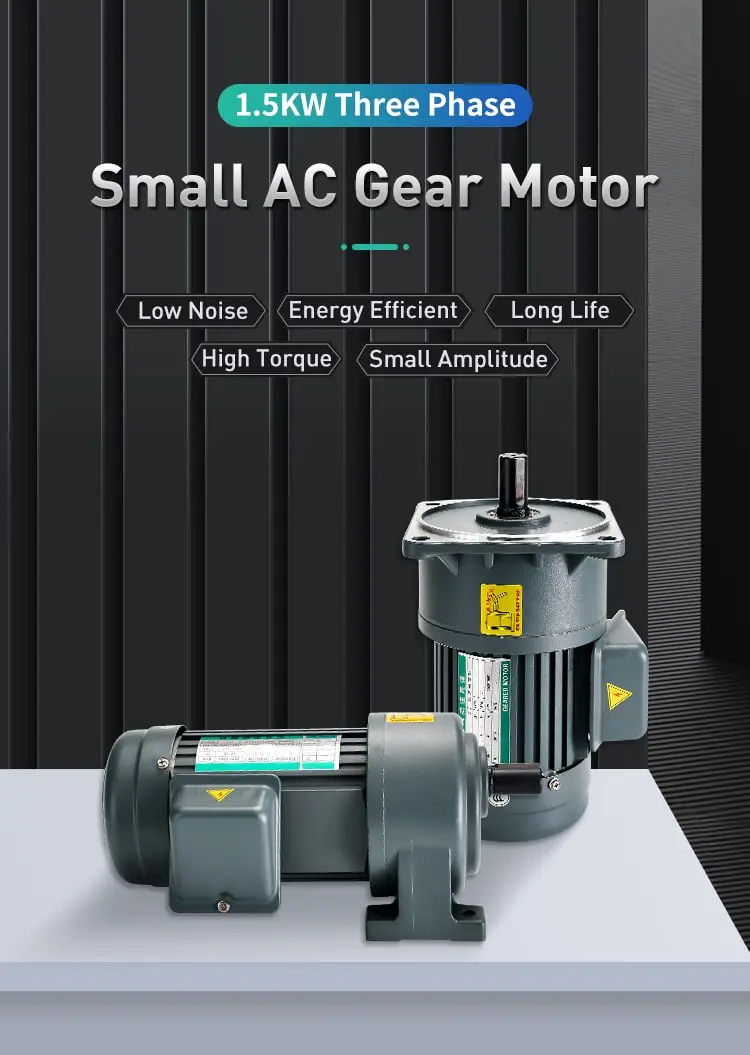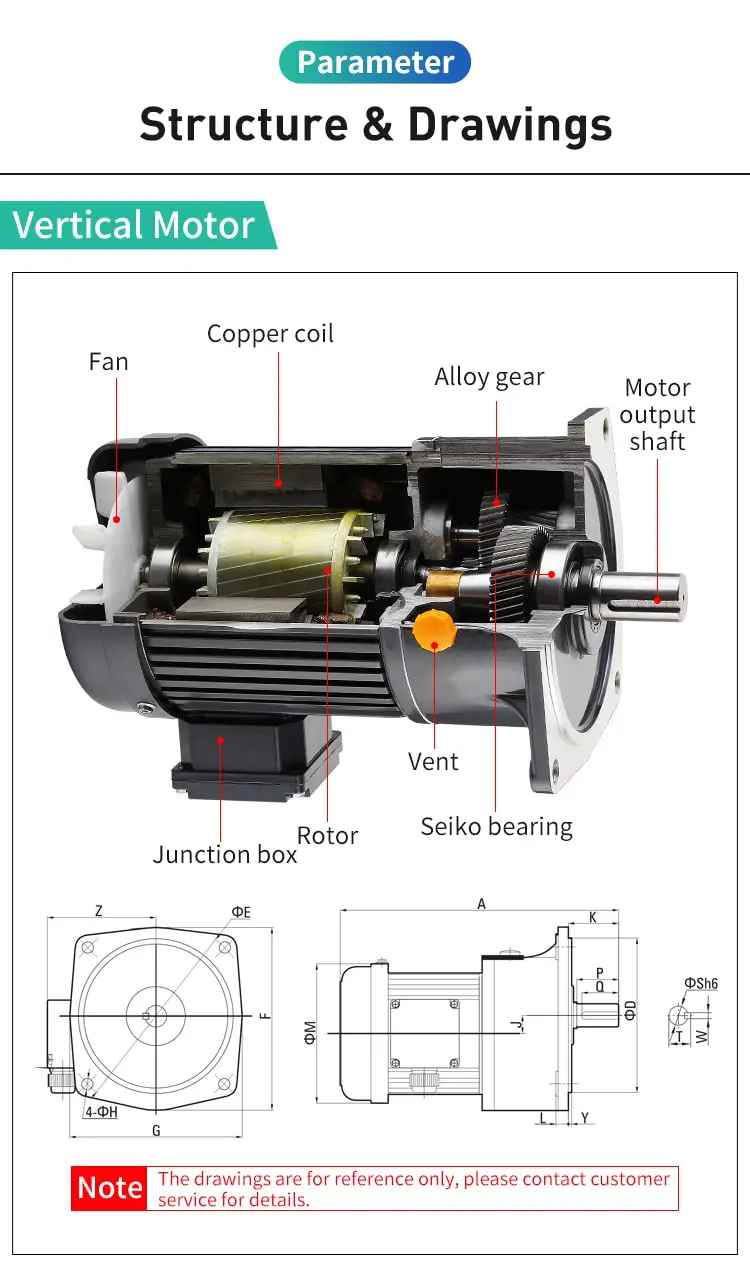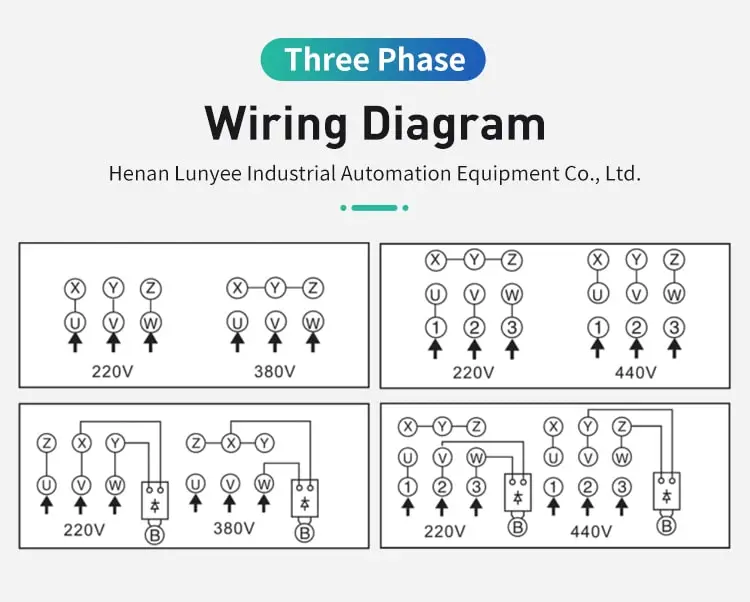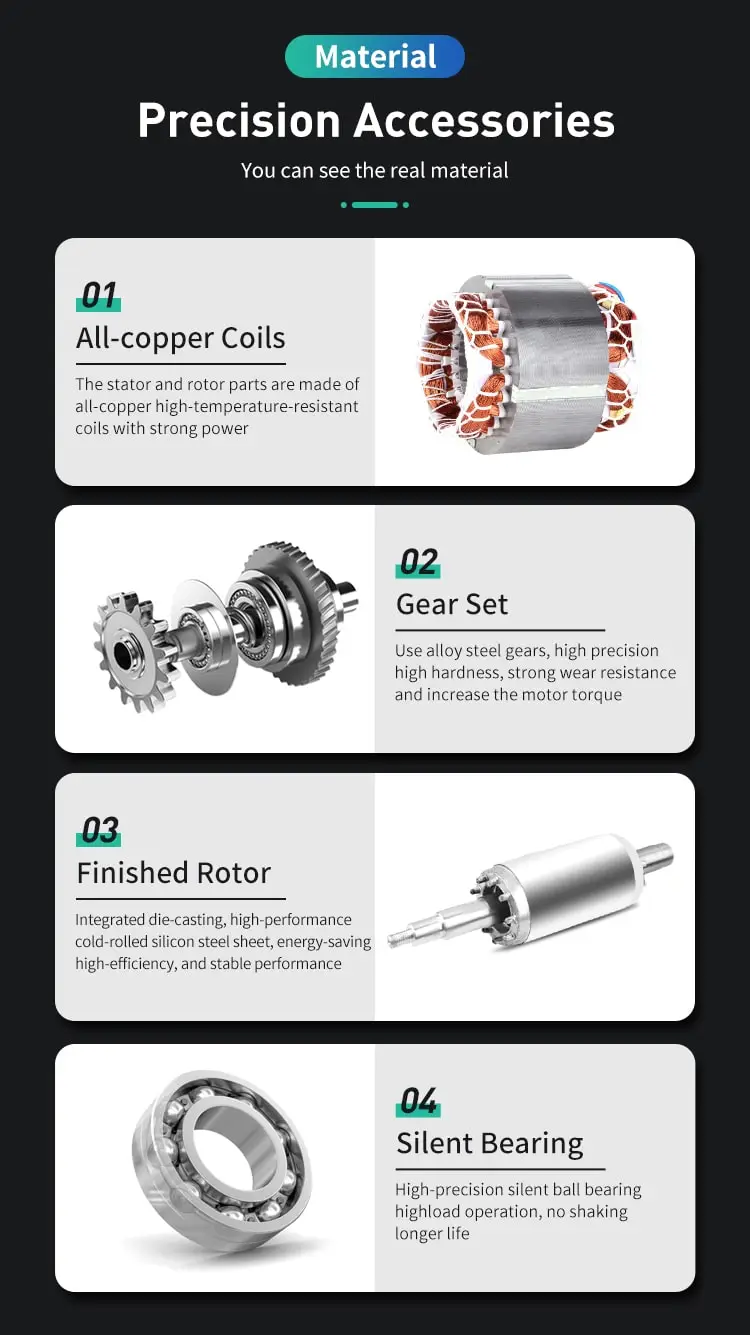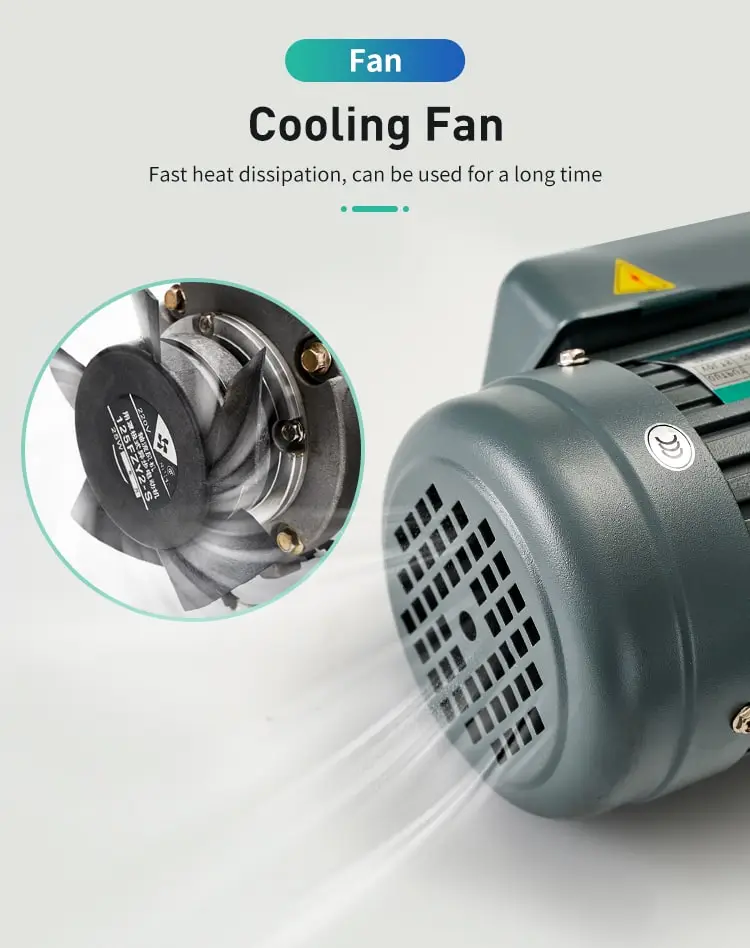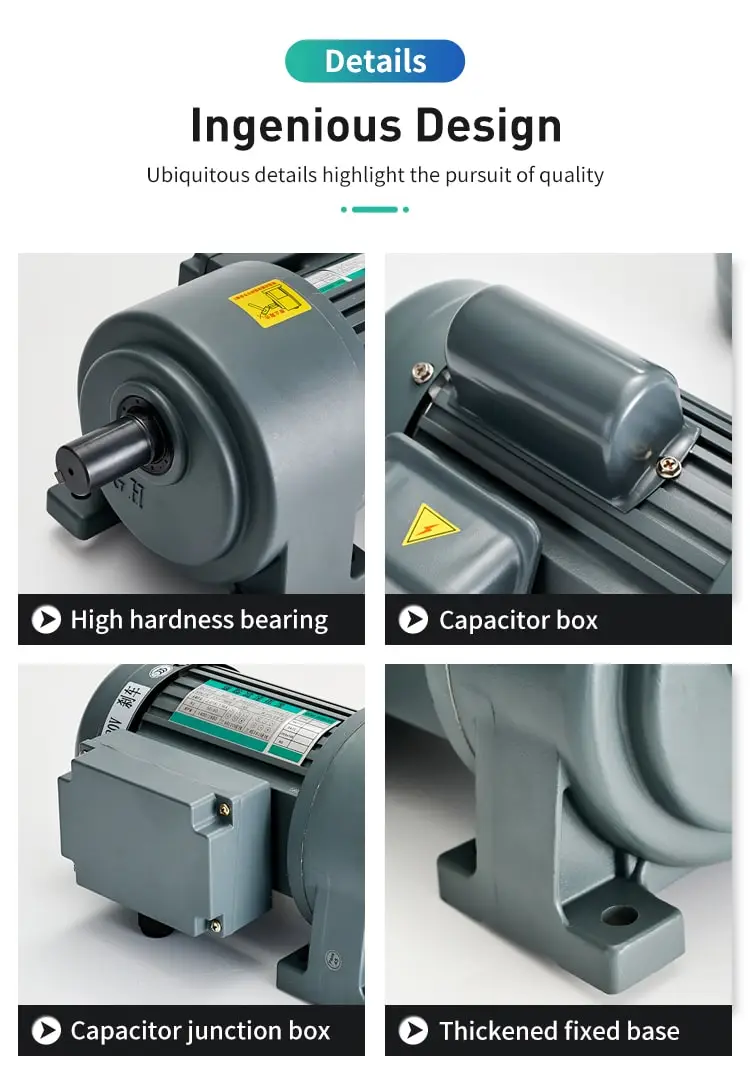By Jasmine 2023-03-10 10:32:17

#### How to measure inductance of a motor?

One way to measure the inductance of a motor is to use an LCR meter or an oscilloscope to measure the voltage and current waveforms and calculate the inductance using the formula L = V / (di/dt), where V is the voltage, i is the current, and di/dt is the rate of change of current. Another way is to use an AC bridge circuit or a resonant frequency method to measure the inductance.

Inductance is an important parameter that characterizes the behavior of electrical devices, including motors. Measuring the inductance of a motor can provide valuable information about its performance and help diagnose issues that may arise during operation. There are several methods for measuring the inductance of a motor, each with its own advantages and limitations. In this essay, we will explore some of the most common methods and discuss their applications.

One of the most straightforward methods for measuring the inductance of a motor is to use an LCR meter. An LCR meter is a specialized device that can measure inductance, capacitance, and resistance with high accuracy. To measure the inductance of a motor with an LCR meter, the motor is disconnected from its power source and connected to the meter using specialized probes or clips. The meter applies a small test signal to the motor and measures the resulting voltage and current waveforms. The inductance can then be calculated using the formula L = V / (di/dt), where V is the voltage, i is the current, and di/dt is the rate of change of current.

Another method for measuring the inductance of a motor is to use an oscilloscope. An oscilloscope is a device that can display voltage and current waveforms as a function of time. To measure the inductance of a motor with an oscilloscope, the motor is connected to a power source and a small test signal is applied. The oscilloscope is then connected in series with the motor to measure the voltage and current waveforms. The inductance can then be calculated using the same formula as with the LCR meter.

A third method for measuring the inductance of a motor is to use an AC bridge circuit. An AC bridge circuit is a specialized device that can measure inductance, capacitance, and resistance by comparing the impedance of a test circuit to that of a known reference circuit. To measure the inductance of a motor with an AC bridge circuit, the motor is disconnected from its power source and connected to the bridge circuit. The circuit is then balanced by adjusting the values of resistors or capacitors until the voltages at the output terminals are zero. The inductance can then be calculated using the bridge equation.

A fourth method for measuring the inductance of a motor is to use a resonant frequency method. This method involves measuring the frequency at which the motor resonates when a test signal is applied. The inductance can then be calculated using the formula L = 1/(4π^2f^2C), where f is the resonant frequency and C is the capacitance of the test circuit.

In conclusion, there are several methods for measuring the inductance of a motor, each with its own advantages and limitations. The choice of method depends on factors such as the accuracy required, the available equipment, and the complexity of the motor circuit. Whatever method is chosen, it is important to ensure that the motor is disconnected from its power source during the measurement to avoid damaging the motor or the measuring equipment. By measuring the inductance of a motor, engineers can gain valuable insights into its performance and troubleshoot issues that may arise during operation.